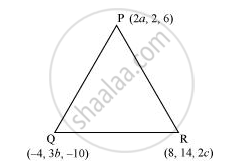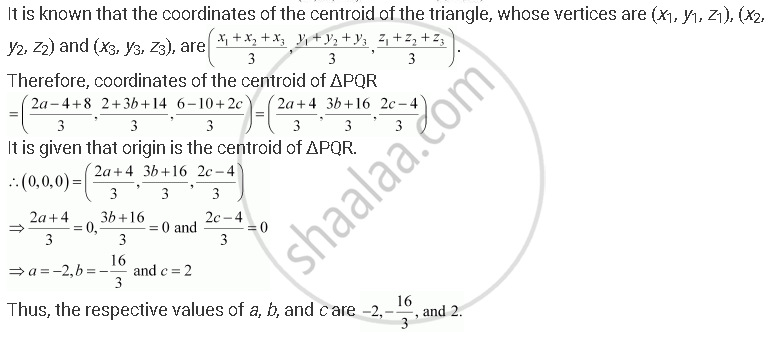CBSE (Arts) Class 11CBSE
Share

# If the Origin is the Centroid of the Triangle Pqr with Vertices P (2a, 2, 6), Q (–4, 3b, –10) and R (8, 14, 2c), Then Find the Values of A, B and C - CBSE (Arts) Class 11 - Mathematics

ConceptThree - Dimensional Geometry Coordinate Axes and Coordinate planes

#### Question

If the origin is the centroid of the triangle PQR with vertices P (2a, 2, 6), Q (–4, 3b, –10) and R (8, 14, 2c), then find the values of ab and c

#### SolutionIs there an error in this question or solution?

#### APPEARS IN

NCERT Solution for Mathematics Textbook for Class 11 (2018 to Current)
Chapter 12: Introduction to Three Dimensional Geometry
Q: 3 | Page no. 278

#### Video TutorialsVIEW ALL 

Solution If the Origin is the Centroid of the Triangle Pqr with Vertices P (2a, 2, 6), Q (–4, 3b, –10) and R (8, 14, 2c), Then Find the Values of A, B and C Concept: Three - Dimensional Geometry - Coordinate Axes and Coordinate planes.
S